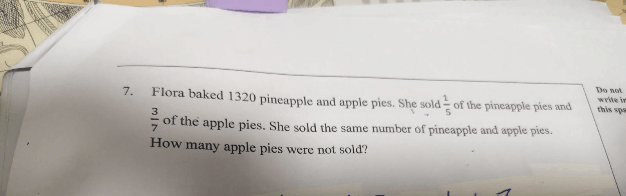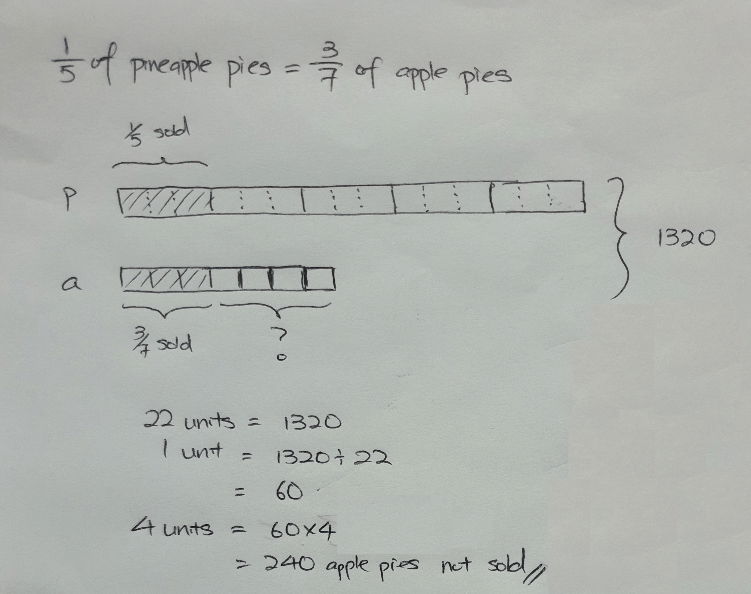# Question0 Replies 1 Like ✔Accepted Answer

1/5x = 3/7 y

(let pineapple pies be x and apple pies be y)

x+y=1320

solve simultaneous equations

0 Replies 0 Likes

let pineapple as X, apple as Y.

We get:

1/5 X = 3/7 Y  (1)

and

X+Y=1320  (2)

Solve the equation to get X=900, and Y=430